# RoadTest Rohde & Schwarz NGL201 Power Supply

Author: ciorga

Creation date:

Evaluation Type: Test Equipment

Did you receive all parts the manufacturer stated would be included in the package?: True

What other parts do you consider comparable to this product?: A power supply is a fundamental instrument in a lab and there are a large variety of power supplies manufacturers. Each power supply comes with a specific configuration (like for example number of channels and voltage range for each channel), with specific performance characteristics, and with specific built-in functions. Performing a full analysis of these factors and a comparison among different power supplies can be a major activity. While I didn't plan to perform such an analysis in this roadtest, based on my exposure to various power supplies over the past 30+ years I am very impressed of the performance and built-in functions of this R&S NGL201 power supply, and I consider it a great instrument for the functionality and performance offered versus price.

What were the biggest problems encountered?: The biggest problem I have encounter was the connection issues with the NGx Sweep Tool software application. My other R&S NGU401 SMU works well with this software but I haven't been able to make the NGL201 work with the NGx Sweep Tool. Second issue was that I couldn't correlate the recovery time specification with my measurements. I have tried two different experiments, and both gave me similar results: it is a large "spike" overshoot in voltage followed by a long settling time much larger than the 30us specification.

Detailed Review:

Contents:

1. A Short Theoretical Tutorial about Power Supplies

2. Unpacking the Rohde & Schwarz NGL201 Power Supply and First Power Up

3. Getting Started with Measurements

4. Output Voltage Ripple and Noise

5. Recovery Time

6. Programmable Source Resistance

7. Arbitrary Waveform Generation

8. Electronic Load - Constant Resistance Mode

10. Current Load Function of the NGL201 Power Supply

11. Battery Testing with NGL201

12. Measuring the Efficiency of DCDC Converters

13. Testing the Startup of DCDC Converters with the NGL201 Power Supply

14. Conclusions

---------------------------------------------------------------------------------------------------------------------------------------------------------------------------

First, I feel honored to be selected as the road tester for the NGL201 power supply instrument and I want to thank Rohde & Schwarz and Element 14 for selecting me.  The following sections will cover various measurements and experiments using the NGL201 power supply. I will start with a tutorial that covers the fundamental concepts of power supplies.

1. A Short Theoretical Tutorial about Power Supplies

What is a Power Supply

A power supply includes a sourcing function, a measurement function, and clamping/protection for maximum current.  The source function in a simplistic approximation can be viewed as an ideal voltage source in series with a parasitic resistance.  The parasitic resistance is “unwanted” here, and a lot of effort is made during design to reduce the value of this resistance.  The measurement function includes a voltage measurement function and a current measurement function.  The current clamping function limits the output current at a programmed value.  When the power supply enters into this current limit mode, it functions as a constant current source.  The output voltage may continue to increase but the current will be limited to the programmed value.  A simplified diagram of a power supply is shown in the figure below.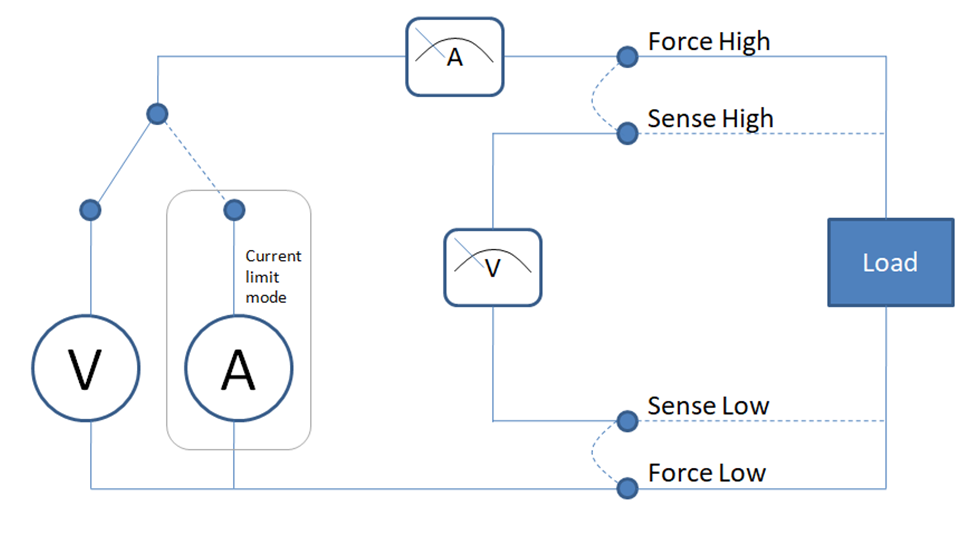The “A” branch symbolizes the power supply functionality in the current limit mode.  The voltage measurement function can probe either the “Force High” and “Force Low” outputs of the power supply or it can probe the external load using two additional wires “Sense High” and “Sense Low”.  The voltage measurement using the external sense wires represents the actual voltage at the load.  The internal voltage measurement represents the voltage at the power supply outputs, which includes the voltage at the load plus the V=IR voltage drops on the wires that connect the external load to the power supply.

Operation modes of Power Supplies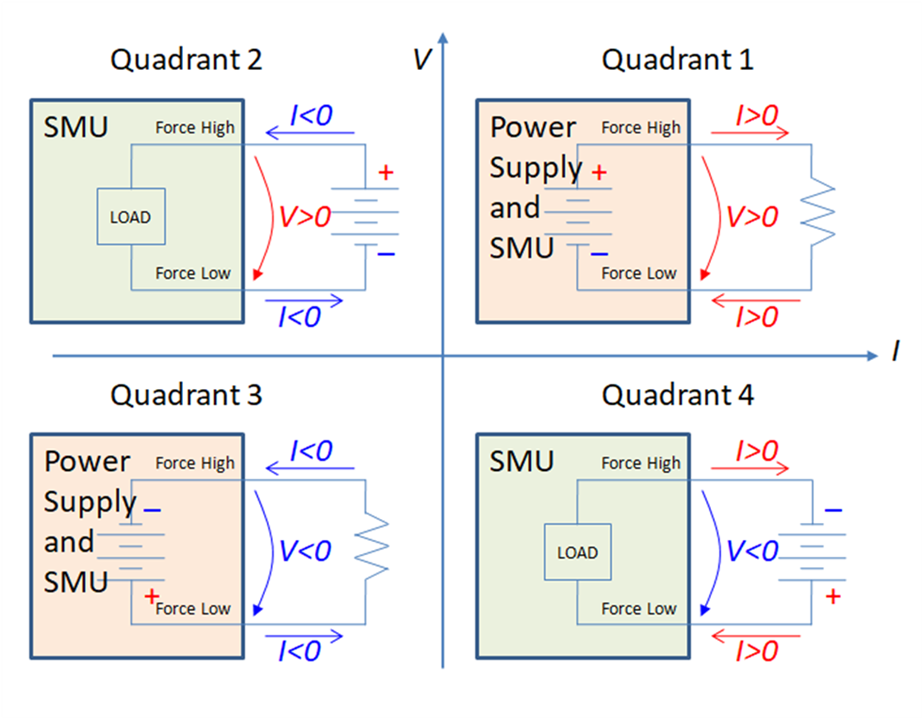For simplicity in this diagram the power supply and SMU are illustrated with two output pins, “force high” and “force low”.  Later the “sense high” and “sense low” pins will be considered in the block diagrams and circuit schematics.  The horizontal axis represents the current flowing out of the force high connector and the vertical axis represents the voltage measured between the force high and force low pins.  Let’s take a look in more details at these operation modes:

A power supply operating in quadrant 1 can function as either a voltage source or a current source.  The power supply operates in force voltage mode when it provides a positive output voltage, and the external load is a passive circuit (no energy sources).  The current flows out of the force high pin, then through the external load, and then it closes the loop by flowing back into the power supply through the force low pin.  When the power supply enters in current limitation or clamping mode, it functions like a current source.  In this mode the current is constant at the programmed limit/clamp value and the voltage will vary (at lower levels than the “open” load programmed value.

The operation in quadrant 3 is very similar to the operation in quadrant 1 with the difference that the programmed voltage is a negative value.  Therefore, the direction of the current is opposite, current flows out of the power supply through the force low pin and it flows back into the SMU through the force high pin.

Power supplies cannot function in quadrant 2; only SMUs function in this quadrant. When the external circuit contains energy sources depending on the voltage/current settings in the SMU the electric current may flow into the force high pin of the SMU.  In this case the SMU instrument operates as an electronic load.  Depending on the external circuit and the SMU voltage and current limit settings if the voltage is positive and the current flows into the force high pin the SMU operates in quadrant 2.  We need to notice here that depending on the impedance of the external circuit and the current limit setting on the SMU the SMU may operate in current limit mode (electronic current load) or in resistive load.

Quadrant 4 also applies only to SMUs since power supplies cannot operate in this quadrant.  The operation in quadrant 4 involves external circuit that contains energy sources, and the polarity of these energy sources are so that the electric current flows into the force low pin of the SMU.  The SMU operates as current load or resistive load depending on the voltage and current limit settings.

In the discussion above I have intentionally omitted the sense pins for simplicity.  The sense pins were assumed to be connected to the force outputs of the power supply (or SMU).   The voltage measured by the power supply or SMU was the voltage at the force high and force low pins, as shown in the diagrams above.  In these cases, if there is a voltage drop on the wires to the external circuit under test or load, that voltage drop difference may affect the accuracy of the measurements.  For these cases the power supplies and SMU instruments use sense pins, sense high and sense low, that the user can connect to the pins of the load.  This way, the measured voltage is right at the pins of the load and the voltage drops on the force interconnect wires do not alter the measured values.  The diagram below shows the operation of power supplies and SMUs in the four quadrants including the sense lines.  Notice that the measured voltage is now at the external circuit pins and not at the SMU force high/low output pins.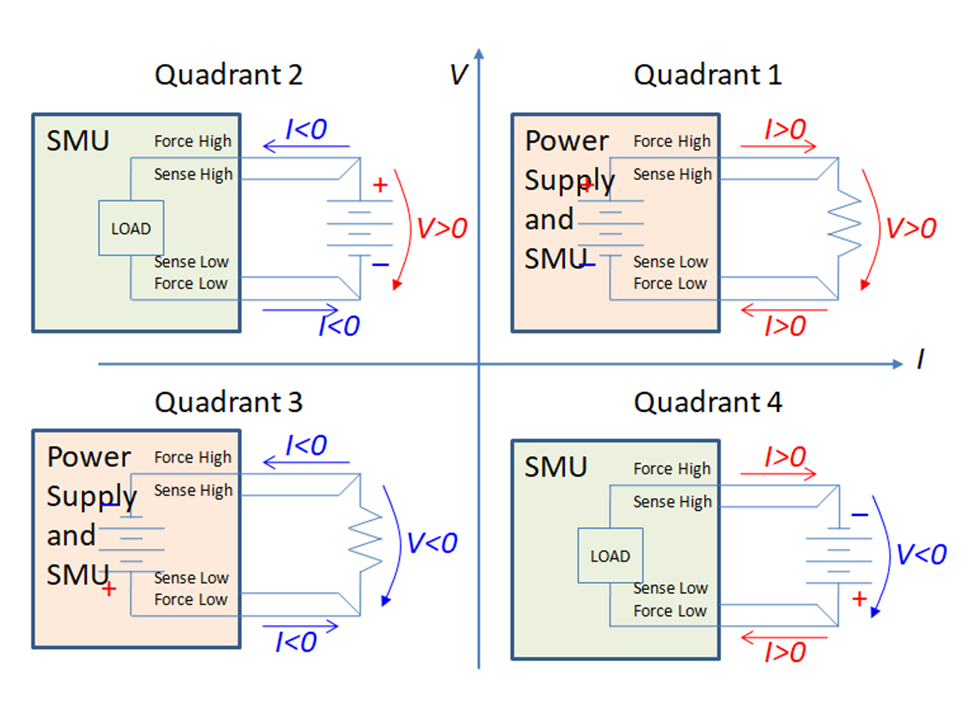Differences between power supplies and SMUs

SMUs integrate the function of a power supply, but they differ from typical power supplies by speed, precision, operation range, and four quadrant versus two-quadrant operation.   SMUs are faster so that typically they settle within microseconds while power supplies settle in much longer time within milliseconds.  This is important in measurements that use sweeping of voltage or current.  The measurement accuracy is significantly higher for SMUs than for power supplies, typically for currents SMUs measurement accuracy is in the order of nanoamperes while for power supplies is in the order of microamperes.  But probably the most important difference is the operation in four quadrants versus power supplies that operate only in two quadrants (quadrant 1 and 3).  This is important for I-V curve tracing where some devices may operate in all four quadrants.  Additionally, this is important for battery testing since batteries can source current and thus may require operating in quadrants 2 and 4.

2. Unpacking the Rohde & Schwarz NGL201 Power Supply and First Power Up

In this section I will describe my experience unpacking and powering up the R&D NGL201 power supply and turning it on for the first time.  The package came announced by an email from Element14, so I knew when to look for it on the porch.  The package was quite big so I have opened it and inside I have found another box which reading on the label it showed that it contains the Rohde & Schwarz NGL201 instrument.  I then opened this box and inside indeed it was the R&D NGL201 instrument packed very well around with a thick polystyrene foam structure, as shown in the figure below.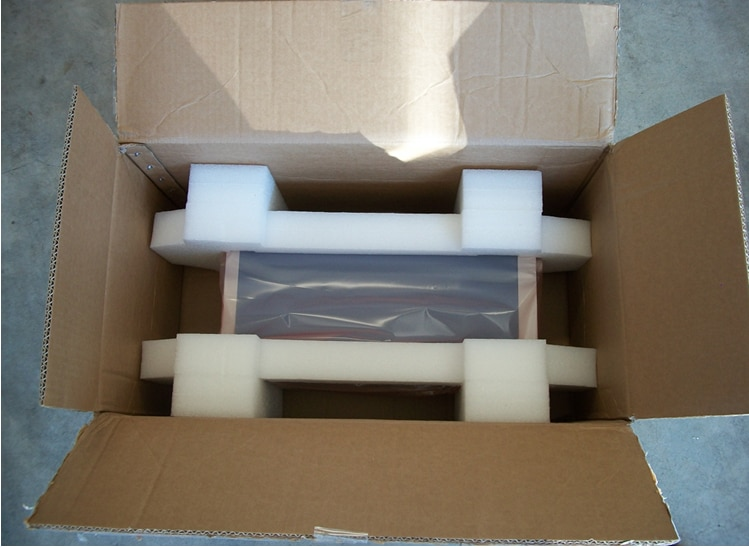A separate box contained a power cable, a getting started guide, and a folder with the calibration certificate and list of license keys.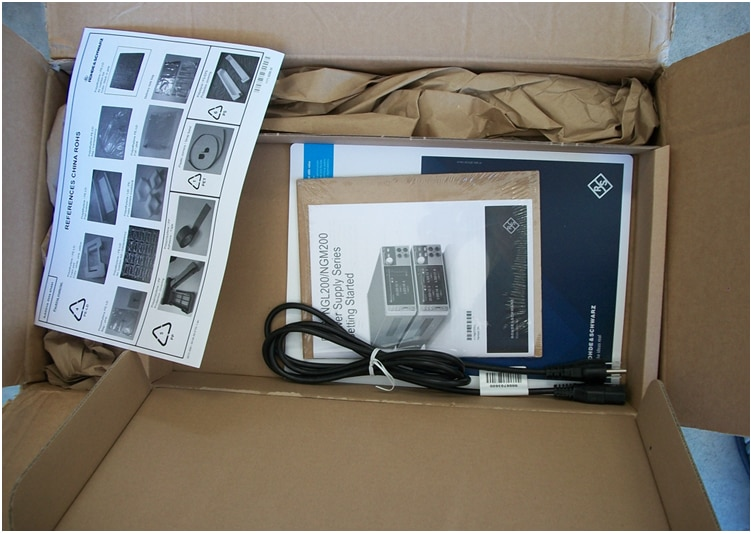The first thing I needed to do was to adjust the settings for the US power voltage standard.  On the back panel of the NGL201 power supply I have noticed a label mentioning that this instrument is set for operating at 230V, which was different than the 120V that I have here in the US.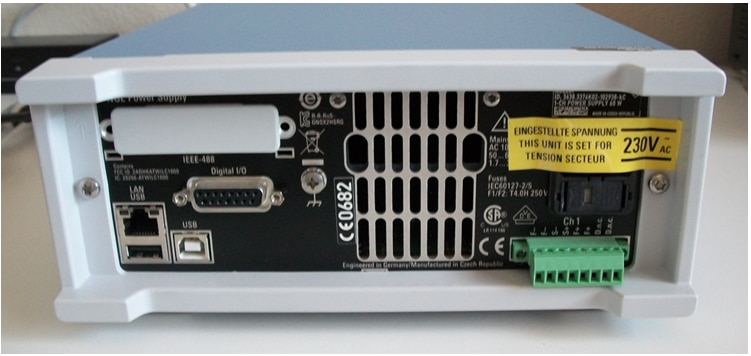I knew how to set up the voltage selector on the back instrument from a previous experience with a similar instrument.  So I started from where the 230V label was and I figured out that if I push on that plastic structure it slides out of the instrument.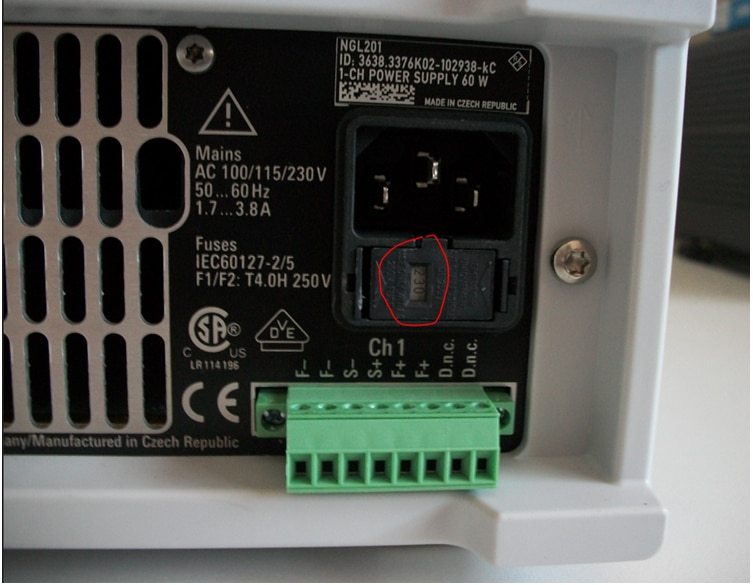So that piece of plastic came out and it held two fuses.  Then, exploring more what came out, I have figured out that it was made of two pieces, one sliding into the other, as shown in the picture below.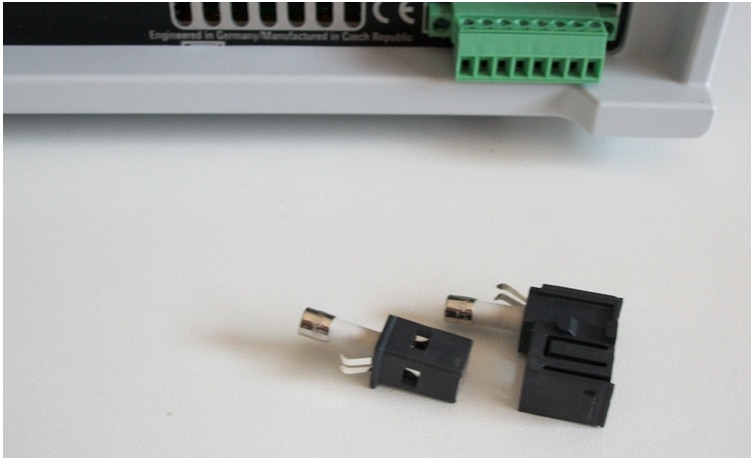After removing the inside piece (that was holding one of the fuses) I noticed three numbers printed on it that were corresponding to the three voltages listed in the getting started user guide.  From this point it was easy – I just plugged the small plastic piece back into the big one making sure that the number 115 shows in the window, as show in the picture below.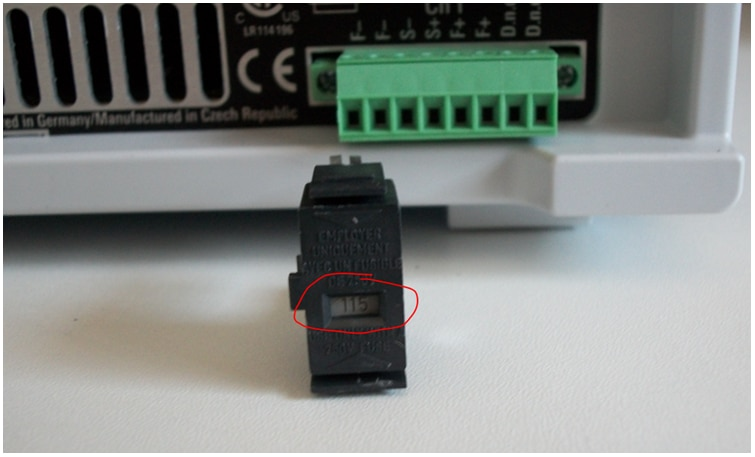And then I have plugged it back into the NGL201 instrument.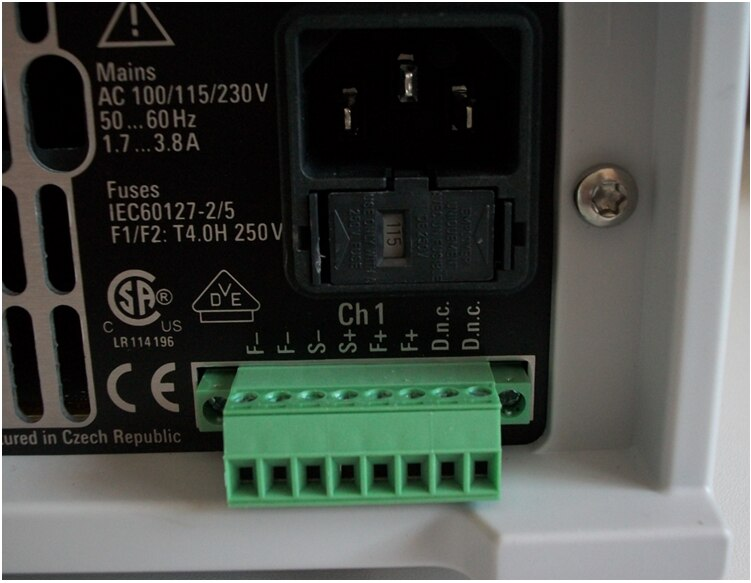Then I have plugged the NGL201 power cable into the power outlet and I pressed the power button – and it worked:

I am now ready to start the next steps of performance evaluation and measurement experiments.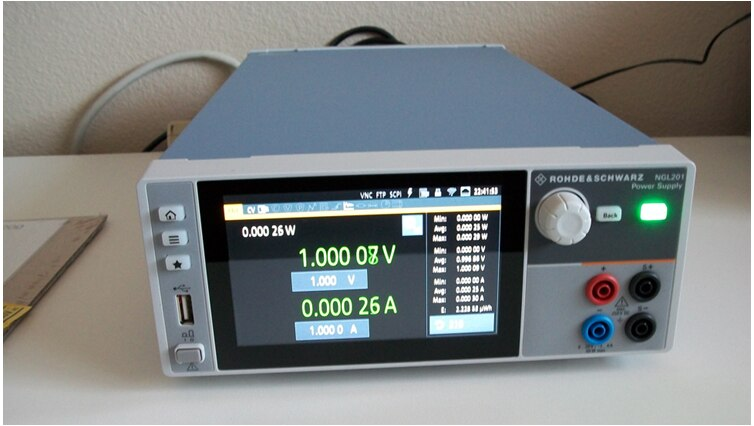3 . Getting Started with Measurements

I have started with a few simple measurements that have setup the R&S NGL201 Power supply to operate into quadrant1 and 3 explained in the first section above.  Here are the details: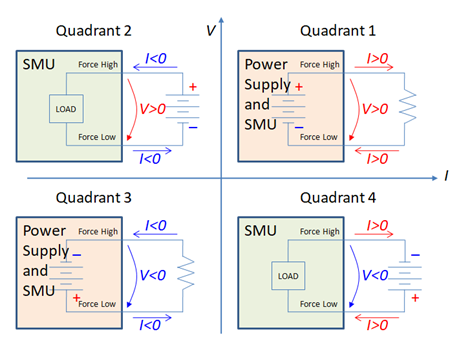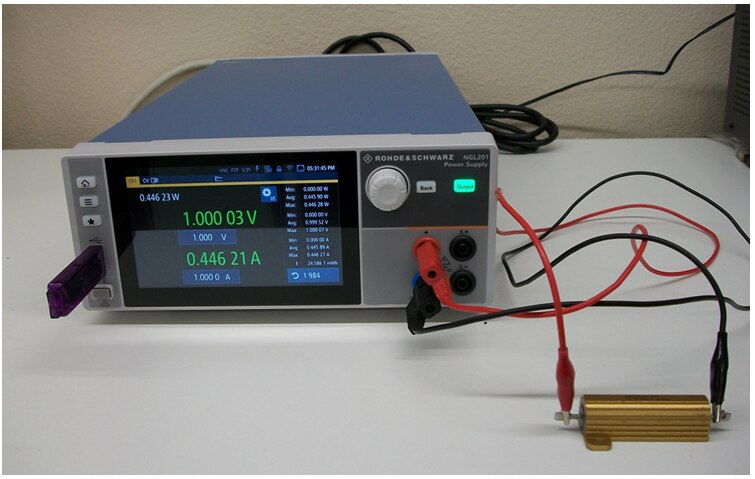For operation in quadrant 1 I have setup the NGL201 to force a positive voltage, 1V, and I connected an external 2 Ohms resistor between the force high and force low connectors.  In this experiment intentionally I did not use the sense lines.  The displayed current was 0.446A while the calculated value was 1V/2Ohms = 0.5A.  The difference comes from the wire resistance, which we can roughly calculate here as 1V/0.446A – 2 Ohms = 0.24 Ohms for both wires, so 0.12 Ohms per each wire.

The operation in Quadrant 3 is setup similarly to the operation in Quadrant 1 with the only difference that the + and – wire connections are reversed so that the voltage applied to the load  has a negative value.  Therefore, the current will also have a negative value, which makes the NGL201 operate in Quadrant 3.

Four Wire Connection

In the measurements above we have noticed that the wire resistance produced voltage drops of about 0.24Ohms x 0.446A = 0.1V, so when we programmed 1V on the power supply the actual voltage applied to the load resistor was only 0.9V.  To eliminate this effect the NGL201 power supply can be configured with 4 wire connection so that the sense voltage is located at the load instead of at the instrument output terminals.  The 4 wire configuration is illustrated in the diagram below: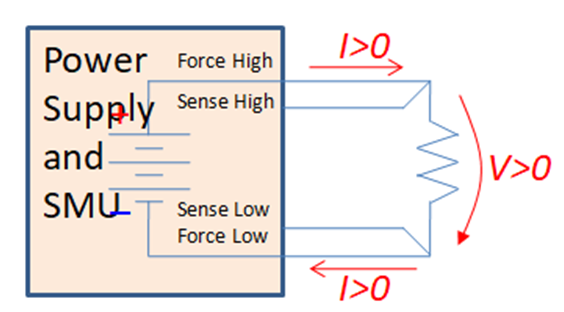And the modified measurement setup is shown in the following figure: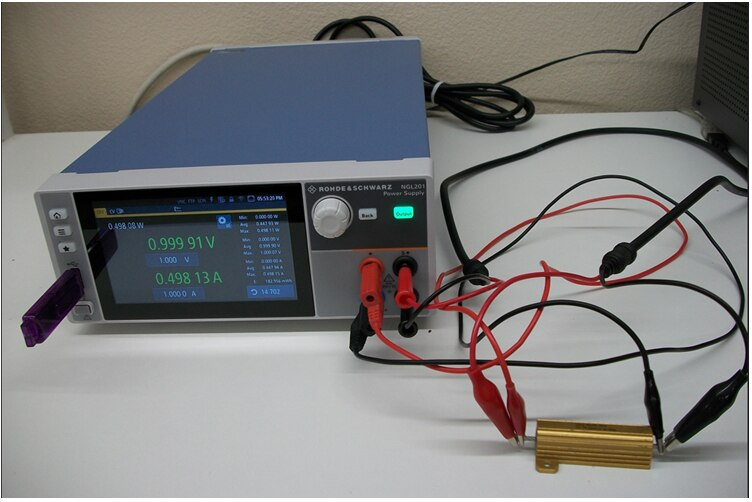Let’s notice now that the current has increased to 0.498A, which is close to the calculated value of 1V/2Ohms=0.5A.  The NGL201 shows a measured voltage of 1V (0.99991V).  This is the voltage at the load.  The power supply has to send a higher voltage to compensate for the voltage drop on the wires, so that at the load the voltage is 1V.  To understand this let’s bring an external DMM and measure the voltages at the NGL201 terminals and at the load.  The DMM measurement at the NGL201 terminals are shown the figure below: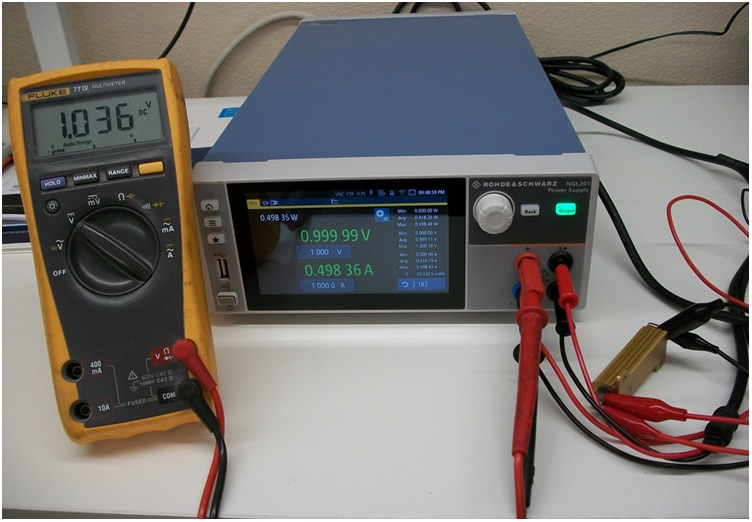The DMM measures 1.036V at the NGL201 terminals.  Let’s move the DMM to the load: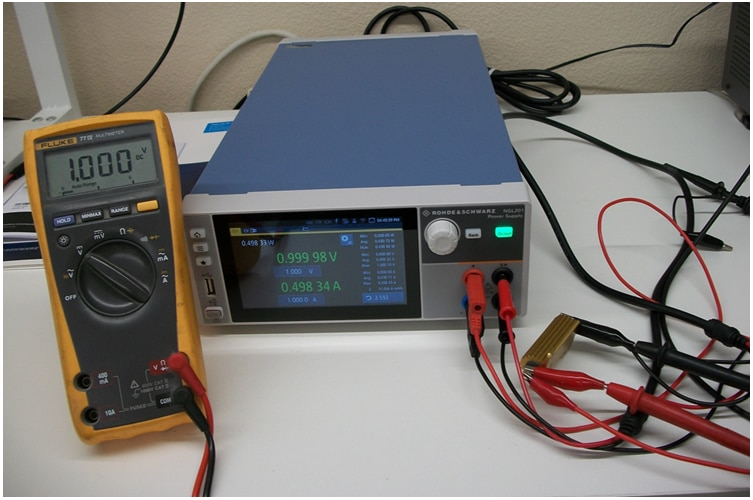The DMM measures 1V at the load.  This value correlates with the reading on the NGL201 screen, which comes in line with the assumption that when using 4 wires connection the NGL201 displays the voltage measured at the load.  So the NGL201 has to send a higher voltage, 1.036V, through the wires in order to have 1V at the load.  The sense lines monitor continuously the voltage at the load and the NGL201 power supply adjusts the voltage at its output terminals so that the voltage at the load is maintained at 1V even when the load current changes.

4. Output Voltage Ripple and Noise

This set of experiments evaluate the NGL201 output noise and ripple. The test setup for measuring the voltage ripple consisted of a 2 Ohms resistor connected as load to the NGL201 power supply in a 4-wire configuration.  The setup is shown in the following figure: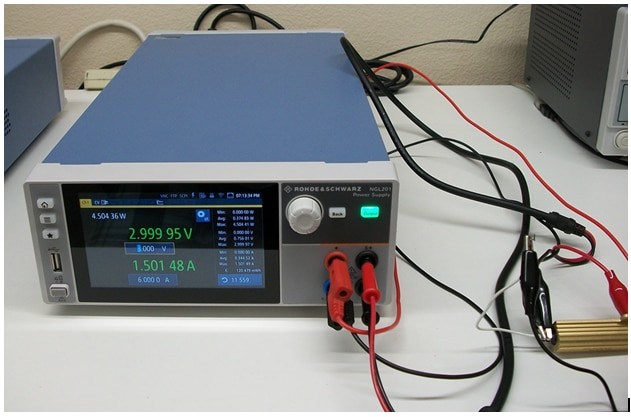The NGL201 has been programmed to 1V, 2V, 3V, 4V, 5V, and 6V. The peak-to-peak and rms ripple has been measured at the load with an R&S RTB2004 oscilloscope. The first set of measurements show very high ripple and noise, so I have further investigated the root cause especially if the measurement setup couples noise from the surroundings.  The first set of measurements is shown in the figures below.  The ripple + noise has been measured using the measure function of the R&S RTB2004 oscilloscope, and it is shown on the screen encircled in the picture with purple colored line.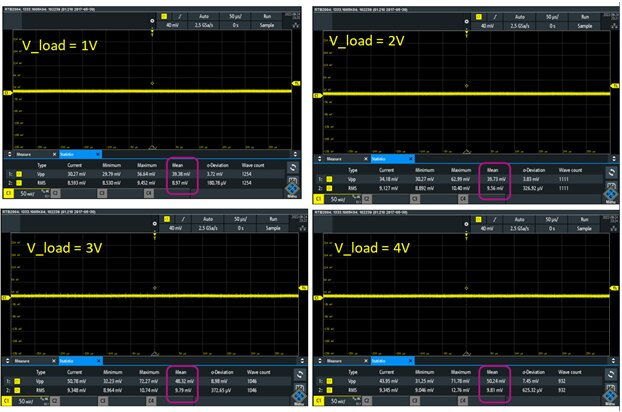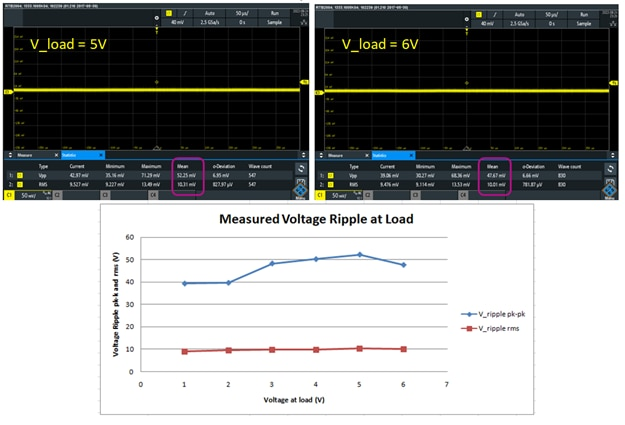The expectation was to see voltage noise + ripple below 2mV pk-pk.  Since the noise levels are much higher than the expected I have looked into any possible noise coupling into the measurement setup.   First experiment was to short the probing clips and measure the noise of the cable only (plus the input noise of the oscilloscope).  Then I have adjusted the previously measured values by subtracting the test fixture noise as rms (as shown in the table):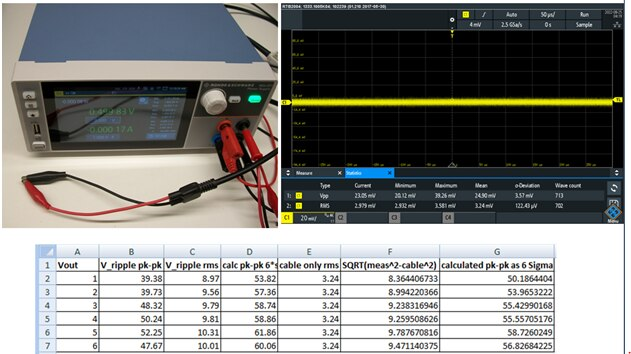The adjustment subtracted the rms noise of the probing cable from the previously measured values (subtracted as rms values, so square root of squares).  The resulted noise (noise + ripple) was still very high, so I decided to remove the resistor thermal noise (by disconnecting the resistor load) and to remove the noise coupling on the wires that connect the NGL201 power supply to the load.  These changes are reflected the setup shown in the figure below: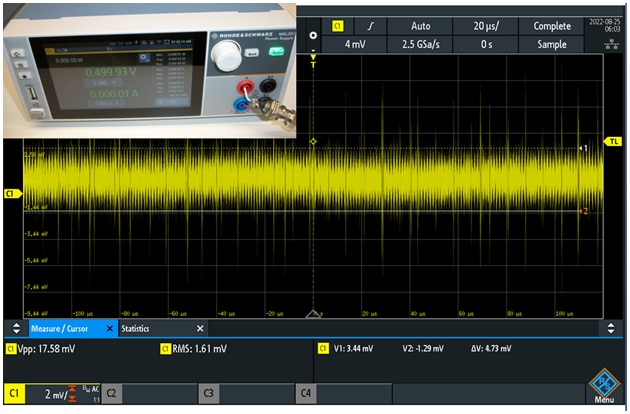The oscilloscope is connected with a coaxial cable directly to the power supply output terminals (with a short wire adapter that I have built myself, and which may introduce some coupled noise but much less than the wires used before).  The waveform shows some “occasional” spikes superimposed on a “more stable” noisy waveform of about 4.73mV pk-pk.  More work to clean up the test fixture got rid of the occasional spikes and left the 4.73mV noise there.  Next, by zooming into the time scale I have noticed that the 4.73mV pk-pk noise has an oscillatory behavior, as shown in the figure below: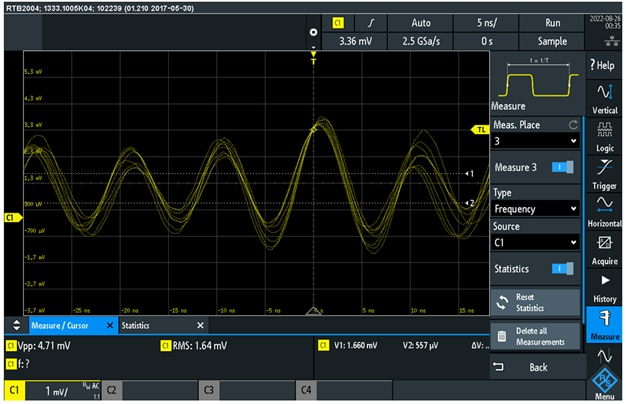To further investigate the oscillatory behavior, I have enabled the FFT measurement function of the RTB2004 oscilloscope, as shown in the figure below: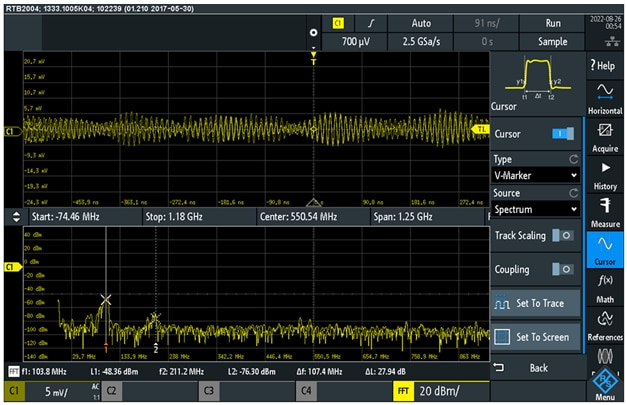We can see in the FFT plot a resonance spur at 103.8MHz and a second one around 200MHz that is probably the harmonic of the first resonance peak.  The resonance peak at 103.8MHz accounts for most of the pk-pk noise.  So I asked myself: is this oscillation on the output of the NGL201 voltage?  To answer this question I have turned the power ON and OFF on the NGL201 power supply.  In both cases, NGL201 with the power ON and with the power OFF the resonance spur at 100MHz was still present as shown in the figure below: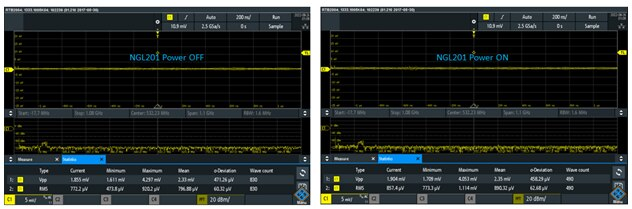The pk-pk mean value is now 2.35mV with power ON and 2.33mV with power OFF.  The rms noise is 0.890mV with power ON and 0.796mV with power OFF.  If I subtract now the noise with power ON from the noise with power OFF as rms values and I then multiply by 6 to get the equivalent pk-pk noise contribution of the NGL201 power supply I calculate:

V_rms = SQRT( (0.890)^2 – (0.796)^2)= 0.398mV  Now if I multiply by 6 to get the pk-pk value: V_pk_pk= 6* 0.398mV=2.38mV  This value is more in line with the spec of 2mV pk-pk.

So I have learned in this experiment that the measurement fixture may have a significant impact in the measured noise and ripple.  The coupling from the lab environment (primarily electromagnetic fields) can alter the measurement to values much higher than the actual levels.

5. Recovery Time

This set of experiments evaluate the NGL201 recovery time.  The recovery time characterizes how fast a power supply responds to a step in the load current.  Here is the definition shown in the NGL201 datasheet: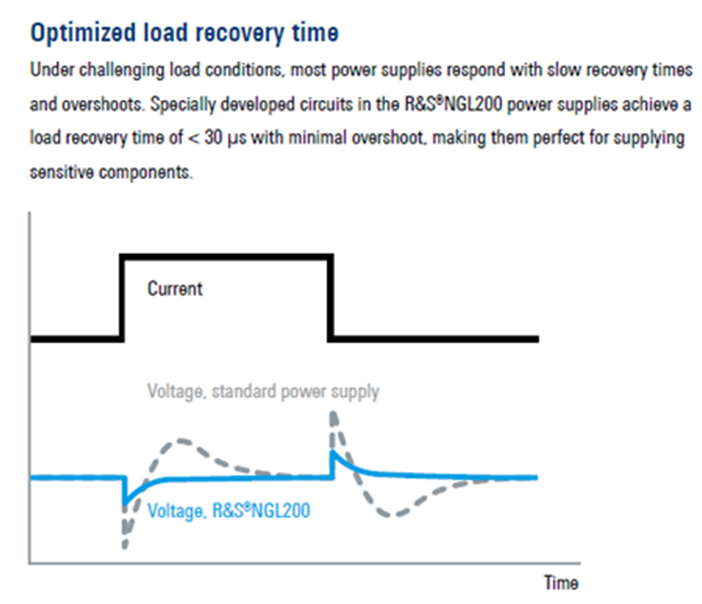The NGL201 power supply has two settings for the transient response: “normal” and “fast” transient response, so I have evaluated the recovery time for each of these settings.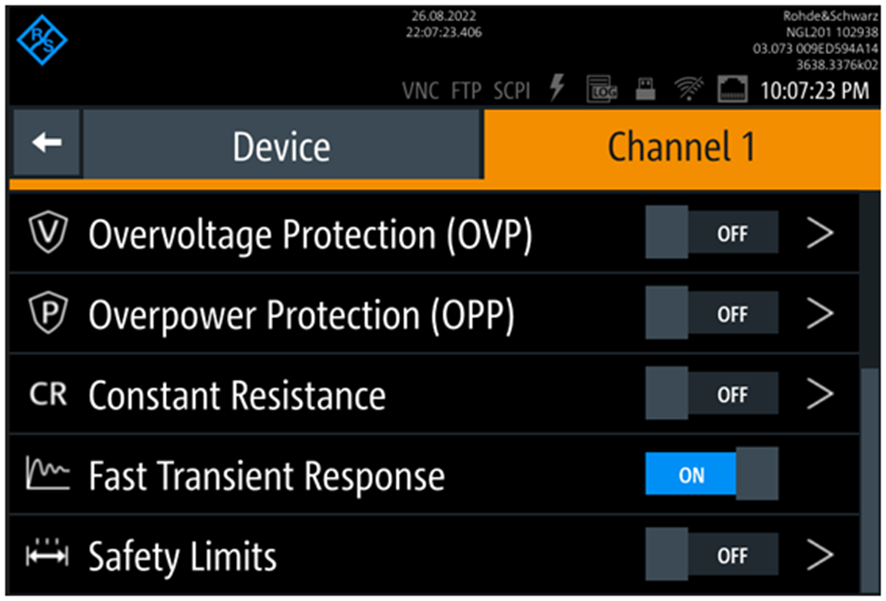For the measurement setup I needed a load that can switch On and OFF periodically, so that I could use and oscilloscope to probe the output voltage and to measure the recovery time.  I decided to use an R&S NGU401 SMU as load, which I have programmed as current load that switches every 1s between 1mA and 3A. This setup is shown in the figure below: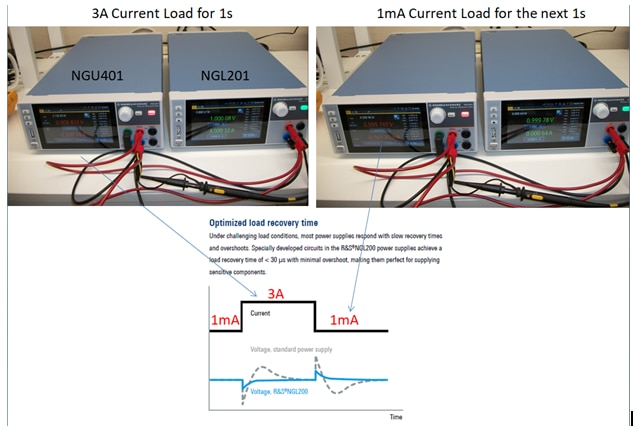The NGL201 was configured in normal and fast transient modes.  The output voltage has been measured with a R&S RBT2004 oscilloscope.  The four waveforms corresponding to rising edge, falling edge, and each for fast transient response and normal transient response are shown in the figure below: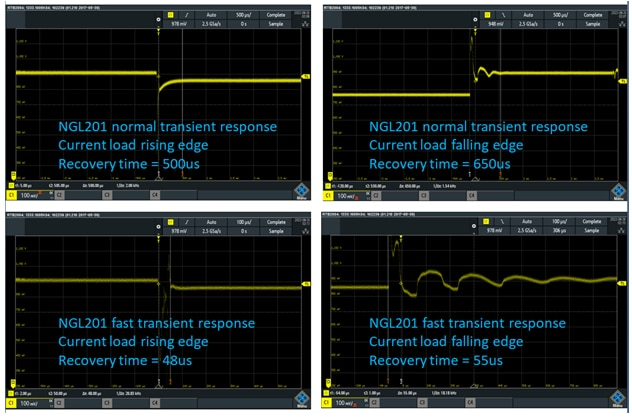In the normal transient response the recovery time is much longer than the 30us spec.  For the current load falling edge I have included the ringing in the probed recovery time.  For the fast transient mode the recovery time is closer to 30us spec but still higher.  In this case I didn’t include the ringing.  Further investigation of the measurement setup and settings could not explain why I am seeing longer recovery time than the specifications.

6. Programmable Source Resistance

The NGL201 power supply has a function that can adjust the source resistance, which can be seen as a resistor in series with an ideal voltage source.  The resistor value can be adjusted from 0 to 10kOHms based on the datasheet specifications.  This section will cover my experiments focused on adjusting the source resistance of the NGL201 power supply.  The adjustment can be accessed from the “Channel” settings menu: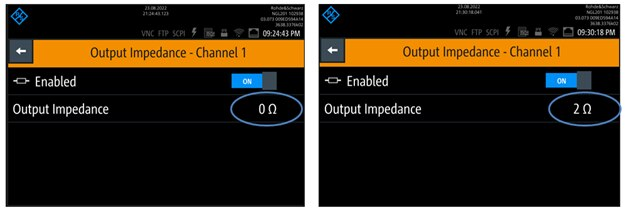The functionality of adjusting the output impedance can be viewed as adding a series resistor to an ideal voltage source.  So the programmed voltage on the power supply is the voltage of the ideal voltage source and the actual voltage that is at the power supply terminals is lower by the V=IR drop on this added source resistance.  The following figure illustrates this model: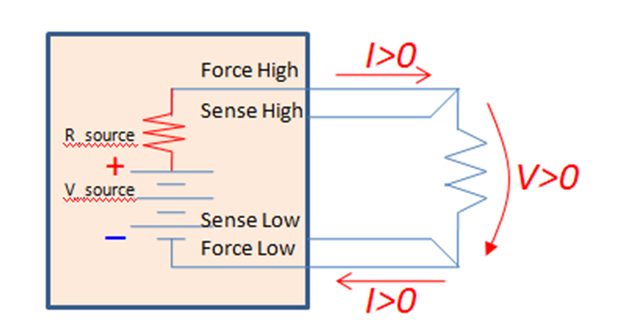To evaluate how the NGL201 has implemented this function I have built the setup shown in the following figure and I have chosen three values for the programmed output impedance: 0 Ohms, 1 Ohm, and 2 Ohms.  The output of the NGL201 is connected to a 2 Ohms resistor load in two and four wires configuration.  For each setting of output impedance I have measured with an external DMM the voltage at the load and the voltage at the NGL201 output terminals.  The figure below shows the measurements fortheNGL201 output impedance set to 2 Ohms.  The programmed voltage is 1V.  The upper two pictures show the 4-wire configuration and the bottom two pictures show the 2-wire configuration.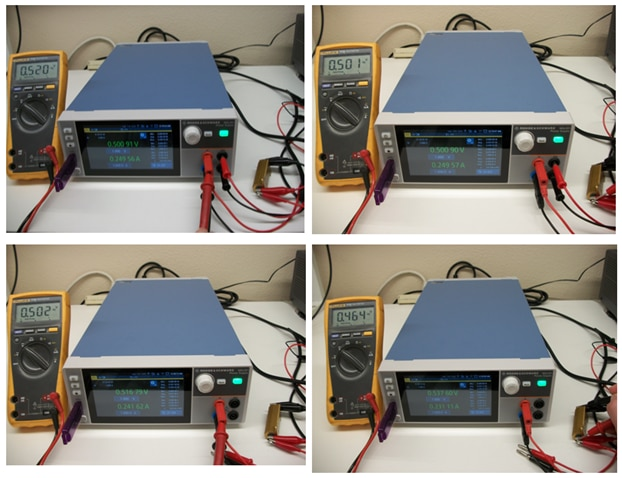The measurement results corresponding to output impedance 0, 1, and 2 Ohms, configuration with2-wires interconnect and 4-wires interconnect are summarized in the table below: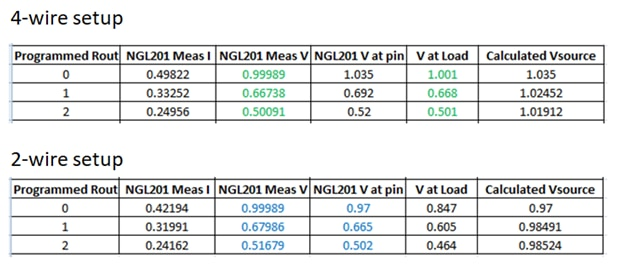Based on these measurements, in the 4-wire configuration the NGL201 displayed voltage is the voltage at the load.  The V_source seems to remain unchanged when R_source takes the values 0 Ohms, 1 Ohm, and 2 Ohms.  It is interesting what voltage reference is used by the voltage regulator control loop since the NGL201 power supply does not know what is the load value.  The programmed value is 1V so is it 1V used as a reference for the control loop?  The sense lines probe the load voltage, which is around 0.5V for R_load=2 Ohms and R_source = 2 Ohms, so does the control loop scale up the sense lines measurement to 1V so that it can be compared with the programmed 1V?

In the 2-wire configuration is looks like the NGL201 measured voltage wants to reflect the voltage at the output pins; however, the measured values with a DMM show quite a significant difference.  So I assume that the displayed voltage represents some internal voltage on the NGL201 output path (because the displayed voltage is a bit higher than the voltage at the output pins)

7. Arbitrary Waveform Generation

The NGL201 can be programmed to generate arbitrary waveforms on the output voltage and current (both when operating as voltage source or as current source).

The arbitrary waveforms can be defined using the waveform generator function available in the settings menu.  The waveform is defined as piecewise linear function with a choice of interpolation (ramp) or raw square wave transitions.  Here is an example for generating a triangular voltage waveform (checking interpolation box makes the transitions in the form of ramps).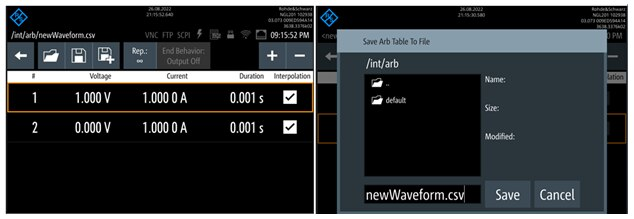After defining the piecewise linear function the waveform needs to be saved, as shown in the right side screen capture.  The saved file is then recalled in  the channel settings when enabling the arbitrary waveform mode: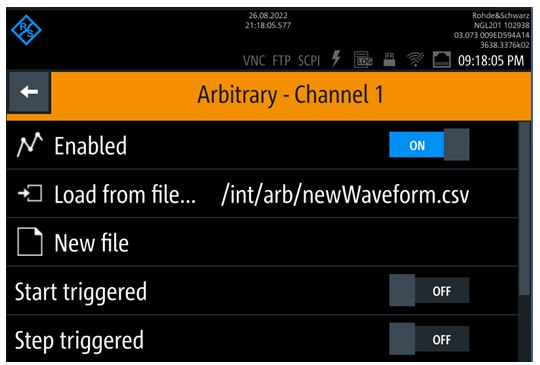The following figure shows two waveforms measured with the RTB2004 oscilloscope at the load (the load is the same 2 Ohms resistor that I have used in the previous sections measurements):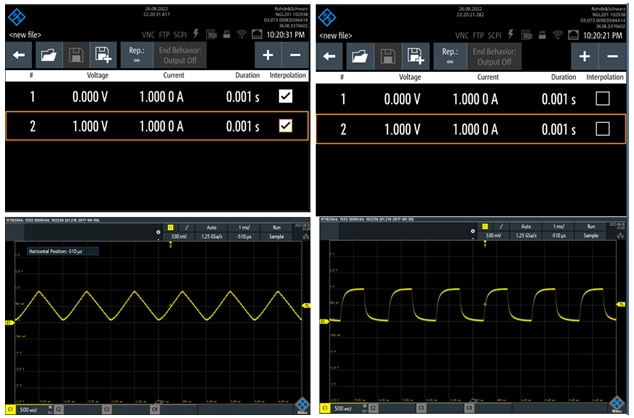The measured waveforms reflect the expected voltage and time values as programmed.  The square wave signal shows a RC low pass type variation on both rising and falling edges.

8. Electronic Load - Constant Resistance Mode

The NGL201 can be programmed as electronic load in three configurations: constant voltage, constant current, and constant resistance.  The voltage and current loads have been seen in the previous sections, so in this section I will look in more details at the constant resistance mode.

Constant Resistance Mode

The NGL201 power supply can be configured to function as a resistor load, and this set of experiments have been focused on evaluating this function.  The experiment setup includes the NGL201 power supply connected as the load to a NGU401 SMU, which is configured as power supply.

The resistor load has been programmed to the following values: 1, 10, 100, 200, 300, 500, 800, 900, 1000, 1100, 1200, 5000, and 10000 Ohms.  For each of these values the voltage and current have been measured and the load resistance has been calculated.  The experimental setup is shown in the following figures: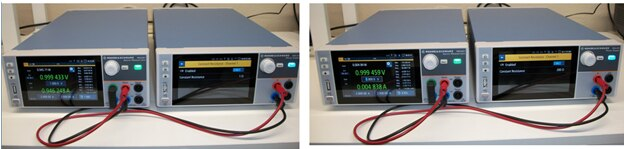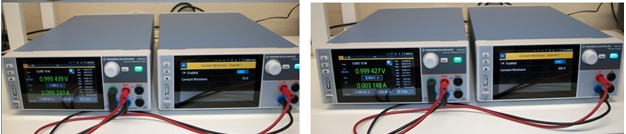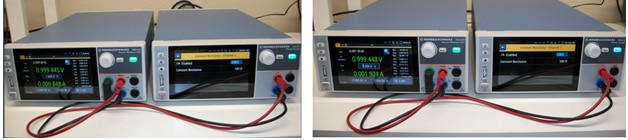The measured values and calculated load resistance are summarized in the table below: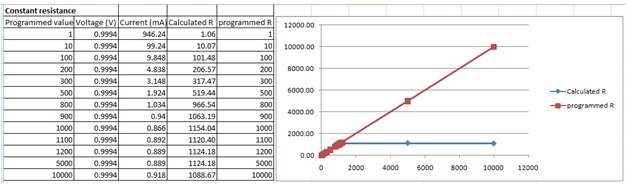We can see in the table and on the graph that the load resistance is limited at 1kOhm.  When the resistance is programmed above 1kOhm, on the spec range 1kOhm to 10kOhms, the actual load resistance of the NGL201 is 1kOhm.

I wanted to make sure that I didn’t miss anything in this experiment setup and I decided to add an external current measure instrument and to repeat the measurements above 1kOhm.  Since my Fluke DMM has the current measure fuse burnt these days (Who didn’t burn the DMM current measure fuse yet?  J ), I have found a good opportunity to feature in these measurements my very first multimeter instrument that my parents bought me while I was in middle school back in 1970’s when they realized that I am so passionate for electronics. The screen glass got cracked recently at the last move, but the instrument still functions.  The reading accuracy is not the same as a DMM but for this troubleshooting experiment it is good enough.

Here are the readings for the NGL201 programmed to 800, 900, 5000, and10000 Ohms: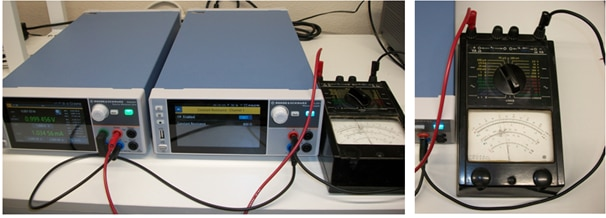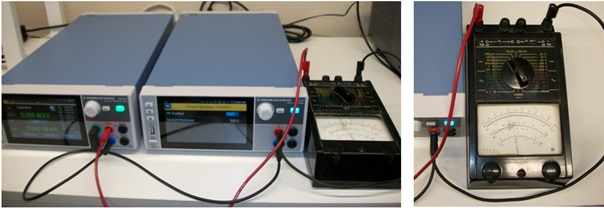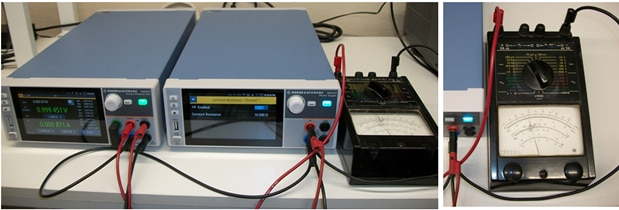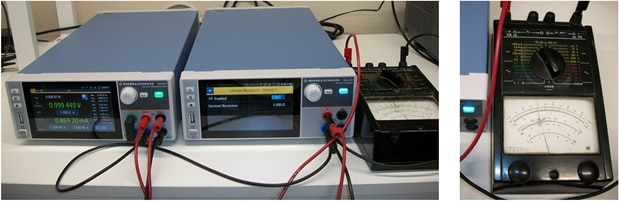The multimeter measured current values agree with the current values displayed by the NGU401 and confirm that the actual current flowing through the circuit when NGL201 is programmed at 5000 and 10000 Ohms are the current values that correspond to the 1kOHm load resistance not 5kOHms and respectively 10kOhms.  So this second part of the experiment confirms that the NGL201 cannot have a load resistance higher than 1kOhm.

The previous experiments that evaluated the NGL201 recovery time showed a much higher recovery time than the specifications, so I decided to try a different test setup to measure the recovery time.  The recovery time characterizes how fast a power supply responds to a step in the load current, and it is specified as <30us for NGL201 power supply.

The NGL201 power supply has two settings for the transient response: “normal” and “fast” transient response, so I have evaluated the recovery time for each of these settings.

In the previous measurements I have used a R&S NGU401 SMU to generate a current load pulse for the NGL201 power supply, and then I measured the transient voltage variations caused by the current rise and fall time transitions.  With this setup there were two instruments involved and I couldn’t tell how much each instrument contributes to the measured recovery time. In this experiment I am using an FPGA to create a current load pulse.  The FPGA is programmed with an IP named “PowerLoad” that makes it draw a constant or pulse current from its power supply.  This PowerLoad IP is intended for FPGAs to test their power supplies on the PCB or in the system.  In this case I have powered up the FPGA from the NGL201 power supply and I configured the PowerLoad IP to generate a current pulse.  I have tried a few settings for magnitude 0.5A and 1A and for frequency 500Hz and 1kHz.  Additionally, I have run the same experiment on the NGL201 power supply and on the NGU401 SMU. The testbench setup is shown in the figure below: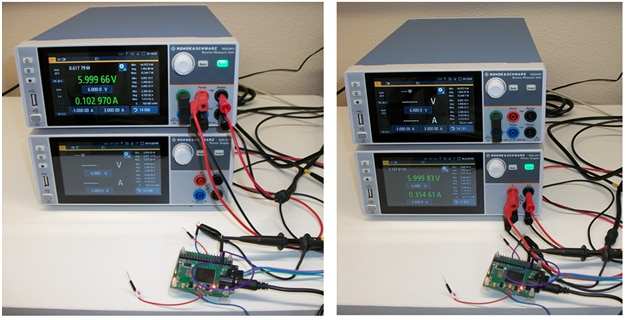The FPGA is on the PCB which is powered from the NGU401 in the left side picture and from the NGL201 in the right side picture.  The PowerLoad software application was running on a computer, and it is shown in the screenshot below: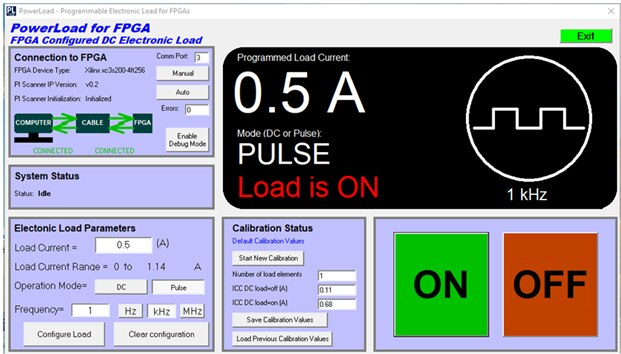The voltage was probed at the sense lines connection to the FPGA in the power distribution network using a R&S RTB2004 oscilloscope.  The measured waveforms are shown in the figure below: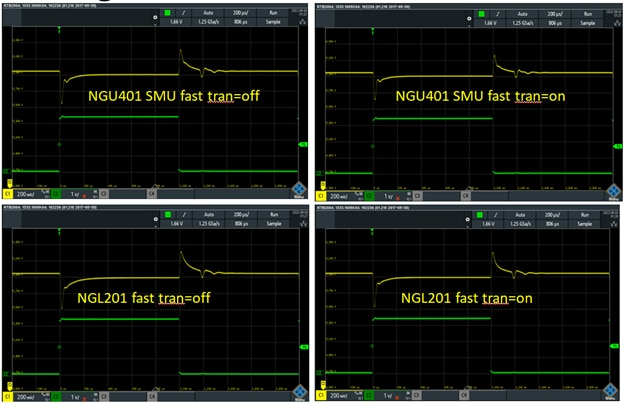These measurements correspond to 0.5A current pulse and the NGL201/NGU401 set to fast transient enabled and disabled.  The yellow trace is the measured voltage, and the green trace is a trigger signal generated by the FPGA.  The trigger signal is synchronized with the current load pulse drawn by the FPGA from the power supply.  The time scale is 200us per division, so we can see that the “complete” settling time is much longer than 30us for both rising and falling edges and in both NGL201 power supply and NGU401 SMU.  These settling time values are similar to the previous measurement when the NGU401 SMU generated the current pulse for the NGL201 power supply.  The following figure shows the recovery time for 1A magnitude of the current load pulse: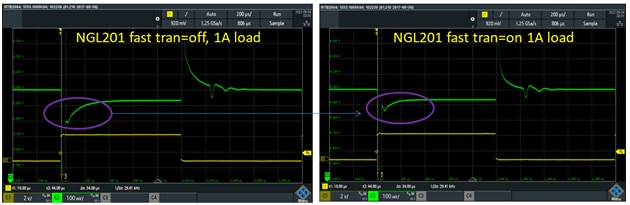I have annotated the difference I see when the NGL201 has the fast transient On and OFF.  The settling time is faster when the fast transient is ON.  Also, the transient response is made of a high magnitude short pulse that is a bit hard to see in the picture followed by a slower speed “RC exponential” type section that is more visible in the picture.  The oscilloscope vertical cursors are set on the short pulse (which here extends almost to the lower and upper edges of the display window), and thus they measure the settling time of the short (high magnitude) pulse.  This measurement shows 34us settling time, which is in line with the NGL201 specification.  Though this short pulse is followed by a long “tail” settling section of more than 200us until the voltage is fully settled.

10. Current Load Function of the NGL201 Power Supply

The NGL201 power supply can be configured as a current load.  The maximum current load and power is defined in the specifications, as shown in the screenshot below: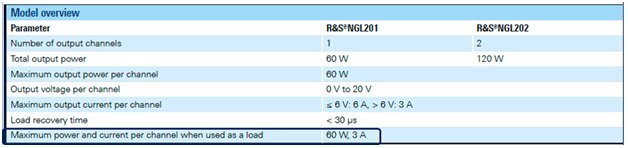In this experiment I have configured the NGL201 as current load and I have connected it to the NGU401, which is configured as a power supply.  The measurement results for various current load settings are shown in the figure below: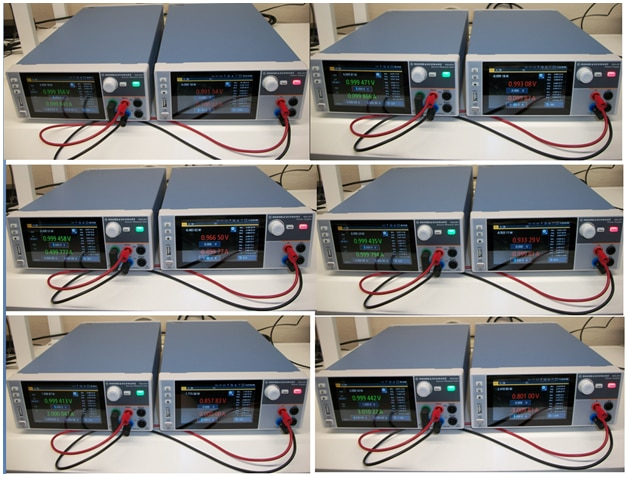The NGL201 current load values have been set to 100mA, 500mA, 1A, 2A, and 3A.  The voltage on the NGU401 has been set to 1V.  This experiment has tested the current load up to the maximum 3A but it has not tested the maximum power of 60W.  The following figure shows the current load measurement with the NGU401 voltage set to 20V and the NGL201 operating at close to 60W power consumption: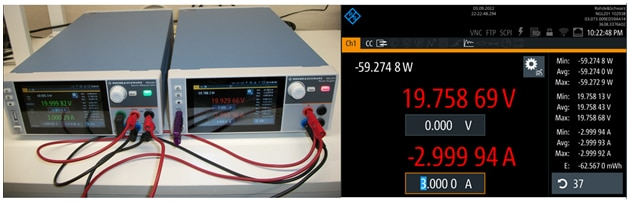The current load experiments showed that the NGL201 meets the specifications as current load without any issues.

11. Battery Testing with NGL201

The NGL201 power supply can be used to measure rechargeable batteries performance.  Typical battery lifetime requirements are within tens of hours (wearable) to ten years (smart sensors).  In this experiment the Rohde & Schwarz power measurement tool for NGL201 will be used to capture the power consumption data and analyze it.

First step I wanted to do but it did not work the way I expected was to install the NGx Sweep Tool on a computer and to control the NGL201 from that application.  The NGx Sweep Tool software is available to download from the Rohde & Schwarz website.  There is also another application available to download named HMExplorer.  So I first installed HMExplorer and that application worked well.  I could communicate with the NGL201 through a USB interface: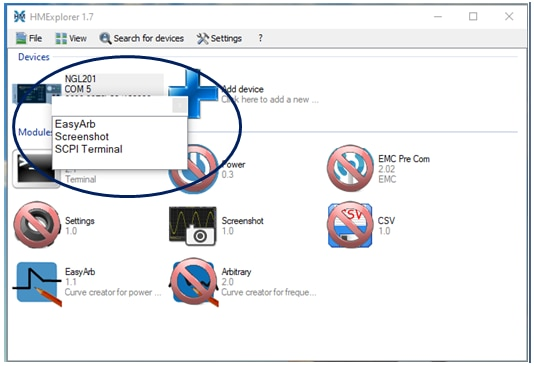But for this experiment I needed to log measurement over time, so I downloaded and installed the NGx Sweep Tool.  The R&S Visa application was also part of the installation: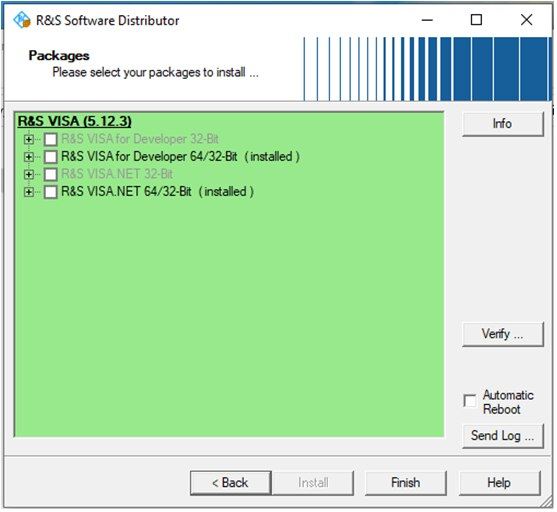The installation completed without any error; however, the NGx Sweep Tool could not connect to the NGL201 instrument.  I have tried another computer with the same results and I have tried to update the NGL201 firmware but is still did not work.  Here is a screenshot with the error message: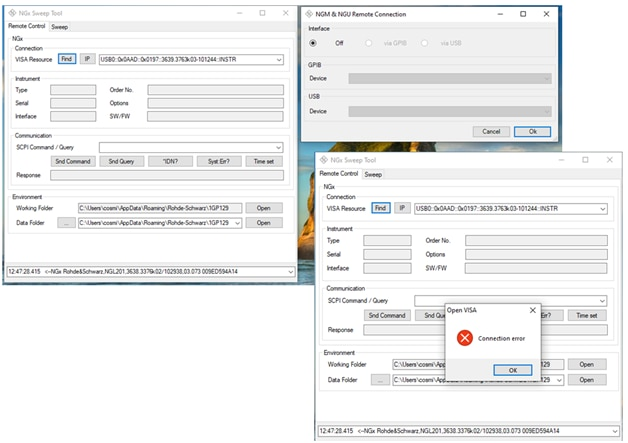I have spent some time debugging the USB connection but I haven’t been able to fix this problem.  Most probably the USB connection works because the other application, HMExplorer can communicate with the NGL201 instrument.  Part of the debugging I tried to connect the NGx Sweep Tool to the NGU401 SMU, and it worked fine: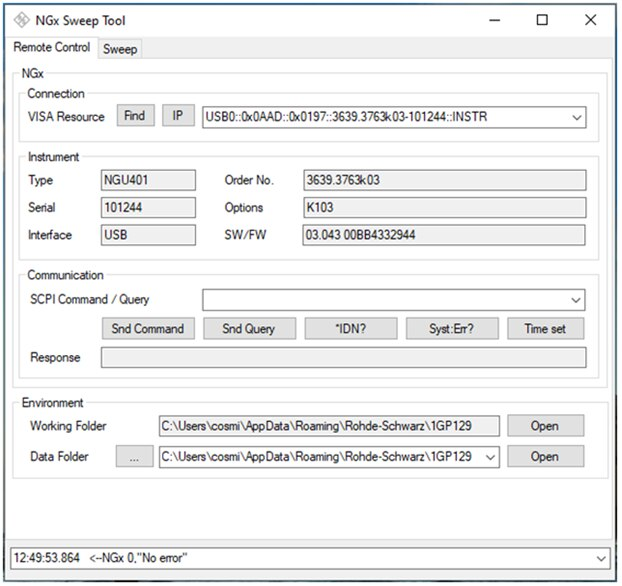So there is some compatibility issue between the NGx Sweep Tool and my NGL201 power supply.

Since the application on the computer did not work, I was left with using the lodging function embedded in the NGL201 power supply.  That logging function saves the measured data in a csv file.  The following figure shows the setup for the .csv file and the logging parameters: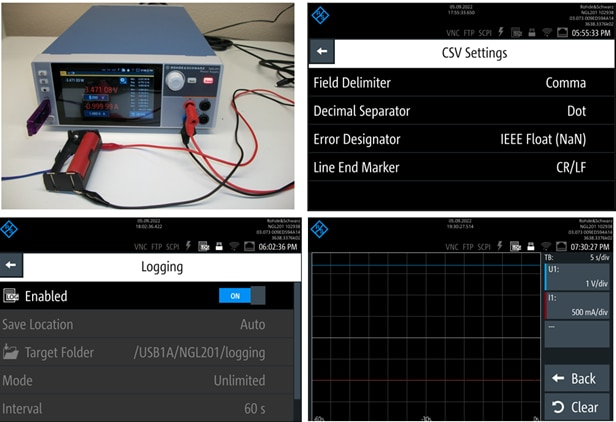This experiment has discharged a Lithium-ion battery with a constant current using the current load function of the NGL201 and the voltage and power have been recorded using the logging function of the NGL201 instrument.  The results are shown in the following figure: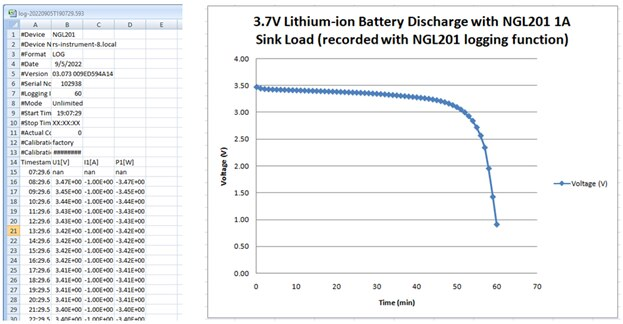The recording time was one hour, and we can see how the battery held the voltage for about 50 minutes after which the voltage dropped rapidly.

The charging back experiment for this battery is shown in the figure below: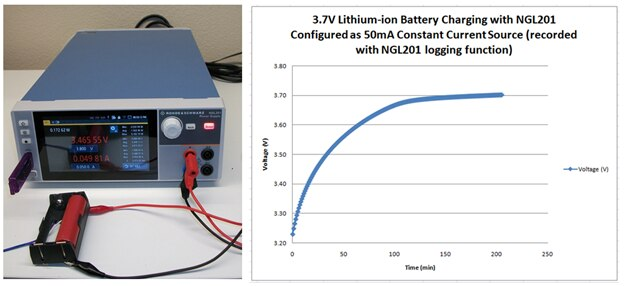The charging current was set to 50mA and the charging time to 3.7V was around 150 minutes.

12. Measuring the Efficiency of DCDC Converters

The NGL201 power supply can be used to measure the efficiency of the DCDC converters.  The measurement needs two power supply channels, so NGL202 would be a better choice for this measurement.  Since NGL201 had only one channel, I have used the NGU401 SMU as the second channel.   The tested DCDC converter was one of the USB car chargers, which has 12V input from the car battery and generates 5V output for a USB powered device.  The setup is shown in the figure below: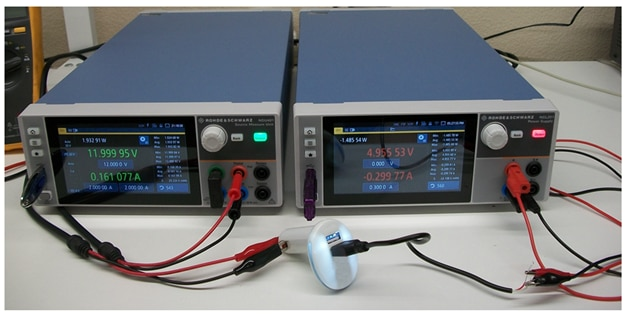The NGU401 is configured as a 12V power supply and the NGL201 is configured as a constant current load.  The measurements at 200mA current load and 1A current load are shown in the figure below: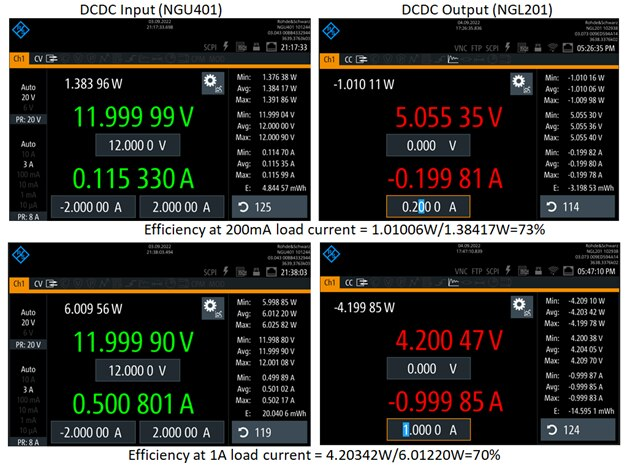The NGL201 and NGU401 display the output power min, max, and average.   Using the average values, I calculated the efficiency as 73% at 200mA load current and 70% at 1A current load, as shown in the figure.

13. Testing the Startup of DCDC Converters with the NGL201 Power Supply

The NGL201 power supply can be used to setup various waveforms for testing the startup of electronic circuits.  In this experiment I have configured the NGL201 power supply to generate different slope ramp type voltages that tested the functionality and performance of a DCDC converter.  In general, slower voltage ramps applied to DCC converters may create high current spikes that may damage the load circuits or the internal circuits of the DCDC converter.

The NGL201 power supply has an arbitrary function generator and also it has a ramp function.  Since I have used the arbitrary waveform generator earlier in this roadtest, for this experiment I decided to use the ramp function.  The Experiment setup is similar to the one used for DCDC efficiency measurement.  The DCDC converter is a car charger that is powered with 12V from the NGL201 instrument and the NGU401 instrument is configured as constant current load.  This setup is shown in the figure below: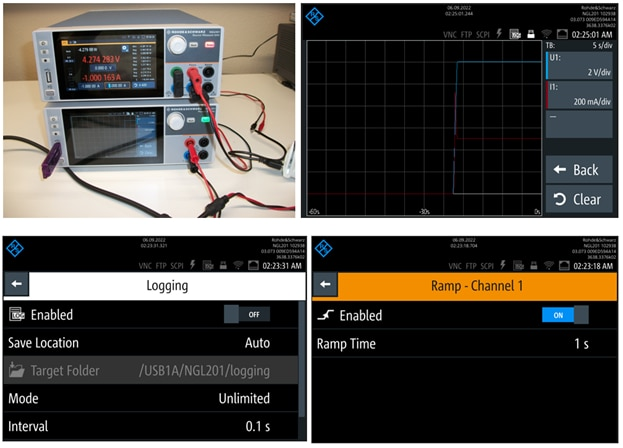The ramp is set to one second and the logging interval to 0.1 seconds, so we expect to see 10 measurement point along the ramping.  The voltage and current variation with time are displayed on the graphic view function of the NGL201 power supply (the blue trace is voltage, and the red trace is current).  Notice that there is a spike overshoot in current during the voltage ramping.  The spike magnitude is about twice the final value.  The voltage and current waveforms have been also recorded by the logging function and they have been saved as a csv file on the memory stick.   The following figure shows the logged data plotted in a Microsoft Excel spreadsheet: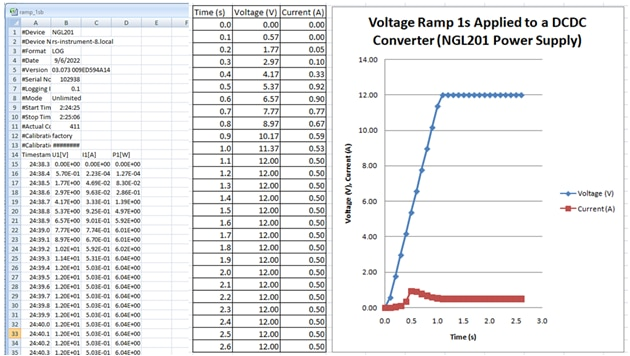The logged values are time, voltage, current, and power.  The graph shows the voltage ramp and the current during this ramp.  The overshoot current spike happens during the first half of the ramp.

I then increased the ramp length to 10 seconds, and I have setup the load current to 0.5A.  The experiment setup and measurement results are shown in the figure below: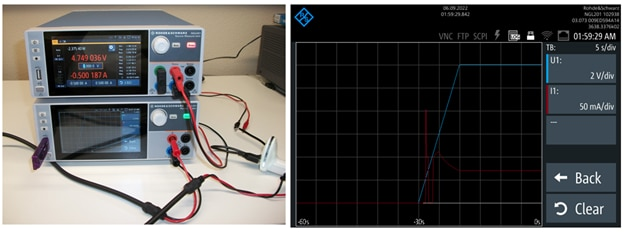The longer ramp time has generated two current spikes: a very sharp one that falls back to zero followed by a “smoother” one which settles at the steady state current consumption level.  Next I increased the current load to 1A, and I performed the same measurement: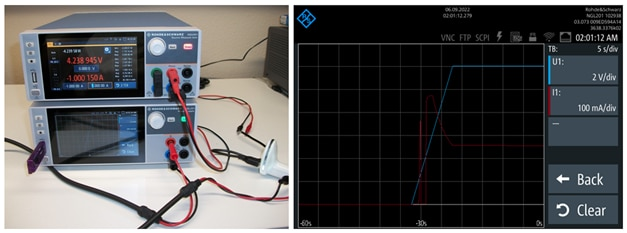There are still two current overshoot spikes.  The first one has similar shape and amplitude like for the 0.5A current load but the second one has a much higher magnitude.  It looks to me that the magnitude of the second overshot scales with the programmed DC current load level.

14. Conclusions

Over the past few weeks I had the opportunity to roadtest the Rohde & Schwarz NGL201 instrument.  I enjoyed this activity and I want to thank Rohde & Schwarz and Element 14 for selecting me as the road testers for this instrument.  During these weeks I have run multiple experiments and I have performed multiple measurements with the NGL201 power supply.  From all this work I am impressed with the quality and performance of the NGL201 instrument.  The front panel display is crisply sharp and clear, and the instrument control is easy and intuitively straight forward.  There were a few performance parameters that I could not correlate with the specifications and some software compatibility problems that I have described above.  My overall conclusion is that the NGL201 power supply is a great instrument made so that it gives me the impression of a solid, high quality, and reliable test equipment.  I feel fortunate for having the opportunity to explore the functionality and performance of the R&S NGL201 instrument through this roadtest.

• Great review.

• Thank you for the analysis and suggestions.  I am not sure if the rise time needs to be so fast (10ns) to see a 30us settling time.  The response seems to settle the high amplitude overshoot (and undershoot) within about 30us but the following exponential "tail" is much longer (>100us).  The ringing is shown only on the rising edge, so most probably it is not caused by the inductance of the wires (because in that case it would have shown on both rising and falling edges).  I will keep looking and this recovery time and if get any interesting results I will post another reply to your message.  Thank you again for looking into this issue and posting your analysis and suggestions.

• Thank you Randall for the nice comments and also thank you, Element 14 community, and R&S for selecting me as the roadtester for this product.

• Yes, I would recommend this product.  The issue that I have seen with the recovery time may be due to the two measurement setups that I have tried.  R&S has advertised the <30us recovery time in the brochure and datasheet, so I am sure they have done extensive analysis and testing for this feature.  The other issue that I had with the software compatibility may have multiple causes not necessarily related to the NGL201 instrument.  And even if the cause is related with the NGL201 I believe it can be easily fixed and the fix added to a future firmware update.  There are two USB modes, CDC and TMC.  CDC works with the HMExplorer software but TMC, which seems to be needed for the NGx Sweep software does not work.  The rest of my experience using this power supply was great.  So, I definitely recommend the NGL201 power supply product.

• For the recovery time measurement I can think of two issues: first - is your NGU401 actually fast enough to test a 30µs recovery time? You need a really fast rise in current to measure that. (I usually use a FET driver by a 555 + FET driver to test this, they can go down to 10ns rise/fall time). You would need to measure the actual current waveform with a shunt and a second scope (isolated, potentially). Second: any measurement lead act as inductance, and will introduce ringing into your measurements.

• Many thanks for a comprehensive and (what looks like) a time-consuming review. I appreciate your effort!

Randall Scasny